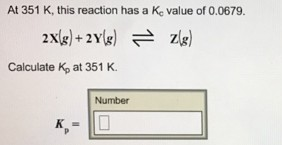# Problem: At 351 K, this reaction has a Kc value of 0.0679. 2X(g) + 2Y(g) ⇌ Z(g) Calculate Kp at 351 K. Kp =

###### FREE Expert Solution
80% (252 ratings)###### Problem Details

At 351 K, this reaction has a Kc value of 0.0679.

2X(g) + 2Y(g) ⇌ Z(g)

Calculate Kp at 351 K.

Kp =